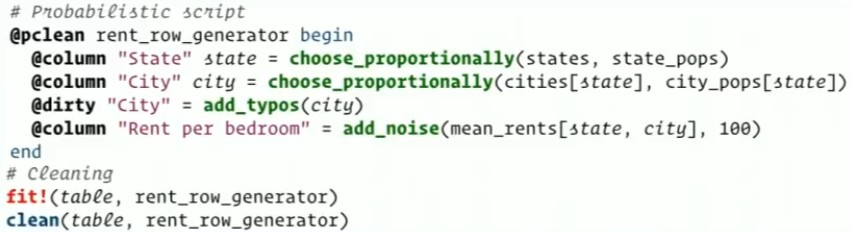# Coin Flipping

A Bernoulli process is a repeated coin flipping, possibly with an unfair coin (but with consistent unfairness).

## Random Variable

$$Y(\omega) = \begin{cases} 1, & \text{if } \omega = \text{heads}, \\[6pt] 0, & \text{if } \omega = \text{tails}. \end{cases}$$

## Sample space

$$\Omega = \{ \text{heads} , \text{tails} \}$$

## PMF

If the coin is a fair coin, Y has a probability mass function given by:

$$f_Y(y) = \begin{cases} \frac 12,& \text{if }y=1,\\[6pt] \frac 12,& \text{if }y=0, \end{cases}$$

## Documentation / Reference

Discover More(Probability|Statistics) - Binomial Distribution

The binomial distribution is the discrete probability distribution of the number of successes in a sequence of n independent yes/no experiments, each of which yields success with probability p. The...Data Mining - (two class|binary) classification problem (yes/no, false/true)

Binary classification is used to predict one of two possible outcomes. A two class problem (binary problem) has possibly only two outcomes: “yes or no” “success” or “failure” and is...Data Quality - Data (cleaning|scrubbing|wrangling)

Data cleansing or data scrubbing is the act of: detecting (ie data validation) and correcting (or removing) corrupt or inaccurate records from a record set, table, or database. Used mainly in...Statistics - Central limit theorem (CLT)

The Central_limit_theoremcentral limit theorem (CLT) is a probability theorem (unofficial sovereign) It establishes that when: random variables (independent) (estimate of a random process) are added...Statistics - Random Variable (Random quantity|Aleatory variable|Stochastic variable)

Random variable is also known as: random quantity, aleatory variable, or stochastic variable A random variable represents the result of a random process. The random variable value is the summary...